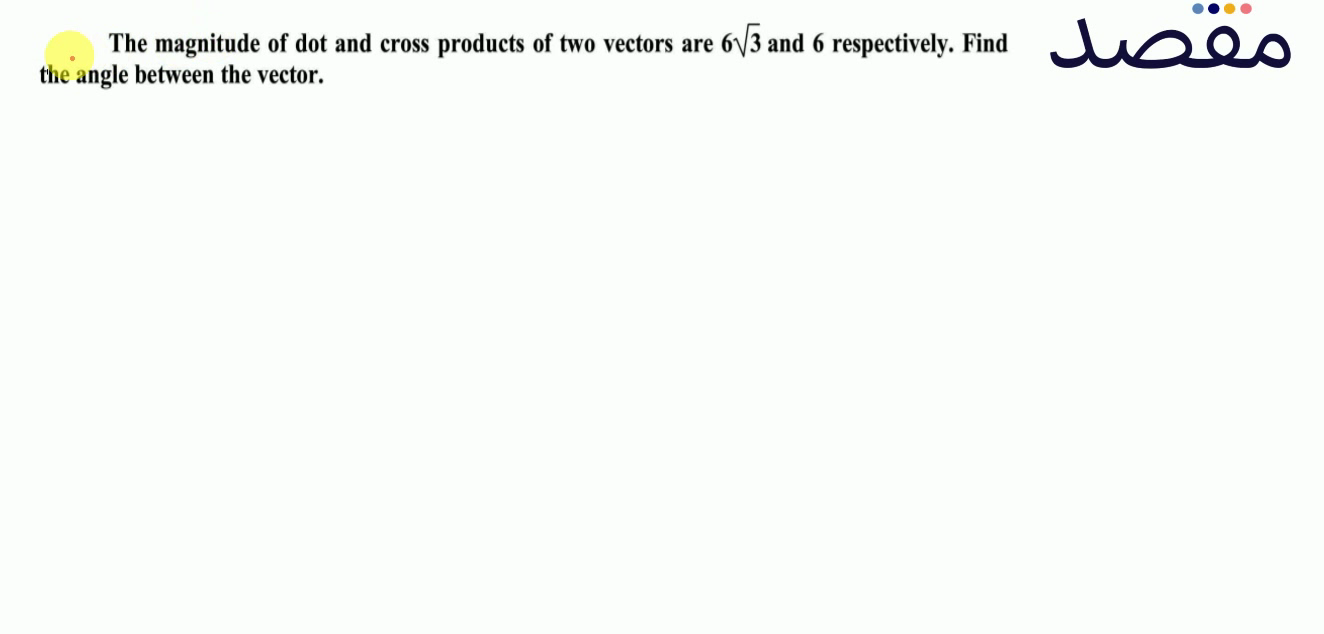# Classes

Change the way you learn with Maqsad's classes. Local examples, engaging animations, and instant video solutions keep you on your toes and make learning fun like never before!

Class 9Class 10First YearSecond Year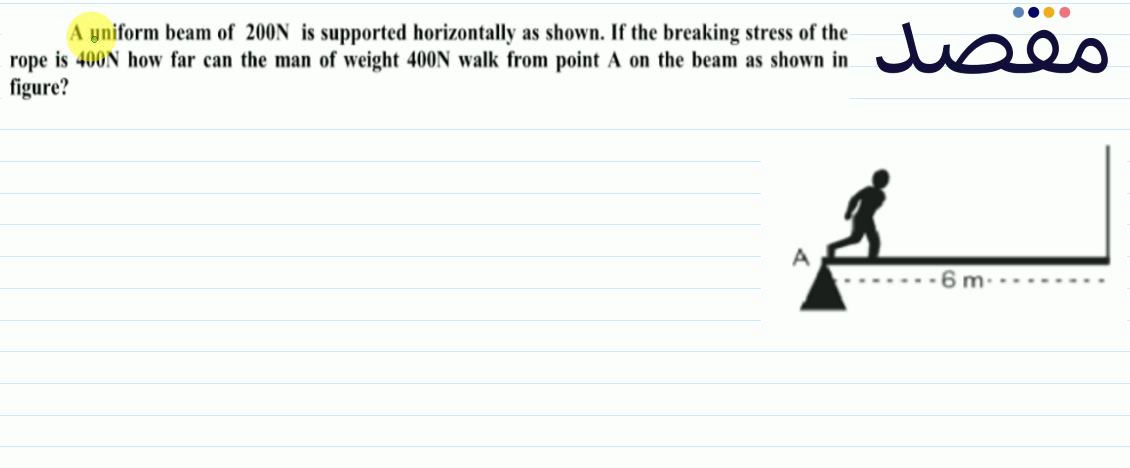### A uniform beam of 200 \mathrm{~N} is supported horizontally as shown. If the breaking stress of the rope is 400 \mathrm{~N} how far can the man of weight 400 \mathrm{~N} walk from point A on the beam as shown in figure?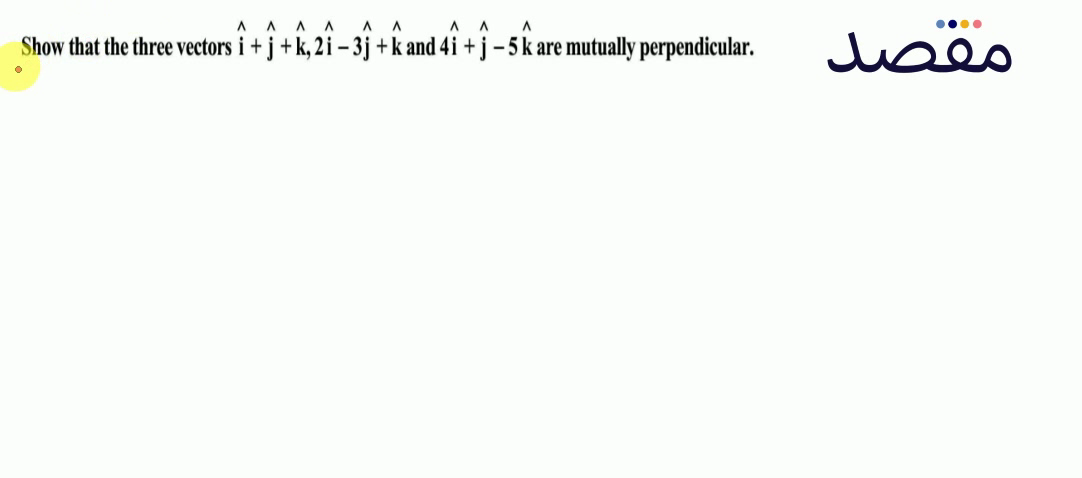### Show that the three vectors \hat{i}+\hat{j}+\hat{k} 2 \hat{i}-3 \hat{j}+\hat{k} and 4 \hat{i}+\hat{j}-5 \hat{k} are mutually perpendicular.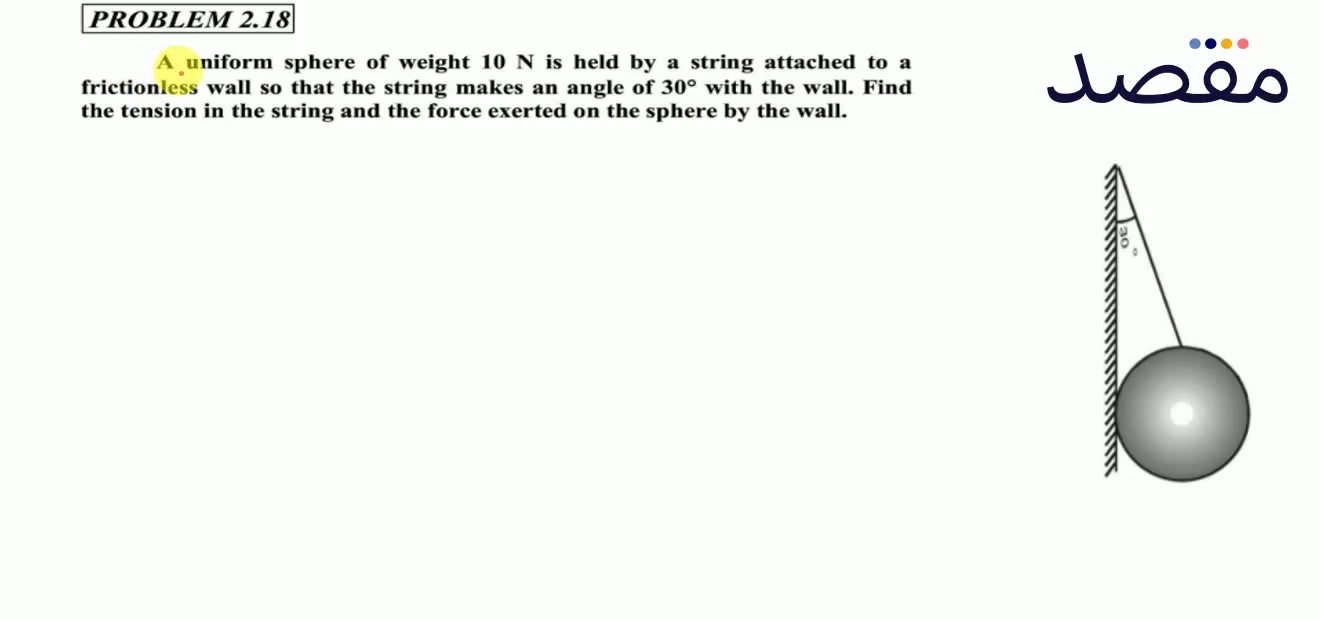### PROBLEM 2.18A uniform sphere of weight 10 \mathrm{~N} is held by a string attached to a frictionless wall so that the string makes an angle of 30^{\circ} with the wall. Find the tension in the string and the force exerted on the sphere by the wall.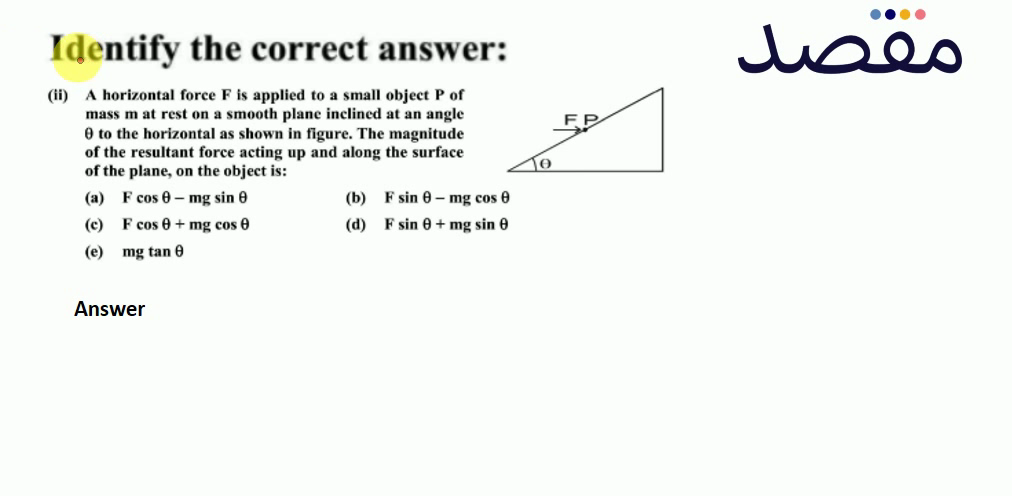### Identify the correct answer:(ii) A horizontal force F is applied to a small object P of mass m at rest on a smooth plane inclined at an angle \theta to the horizontal as shown in figure. The magnitude of the resultant force acting up and along the surface of the plane on the object is:(a) F \cos \theta-\mathrm{mg} \sin \theta (b) \mathrm{F} \sin \theta-\mathrm{mg} \cos \theta (c) F \cos \theta+m g \cos \theta (d) F \sin \theta+m g \sin \theta (e) m g \tan \theta### Show that the sum and difference of two perpendicular vectors of equal lengths are also perpendicular and of the same length.